# Multiplication And Division Of Complex Numbers

Go back to  'Complex Numbers'

Multiplication:

Consider two numbers $${z_1} = {x_1} + i\,{y_1}\,\,{\rm{and}}\,{z_2} = \,{x_2} + i\,{y_2}$$ which we have to multiply. Intuition suggests that we should simply apply the distributive law to accomplish this task.

\begin{align}{z_1}{z_2} &= \left( {{x_1} + i\,{y_1}} \right)\,\, \cdot \,\,\left( {{x_2} + i\,{y_2}} \right)\\\\& = {x_1}{x_2} + i\,{x_1}{y_2} + \,i\,{x_2}{y_1} + {i^2}\,{y_1}\,{y_2}\\\\& = \left( {{x_1}{x_2} - {y_1}{y_2}} \right) + \,\,i\,\,\left( {{x_1}{y_2} + {x_2}{y_1}} \right)\;\;\;\;\;\;...(2)\end{align}

For example:

$$\left( {3 + i} \right)\left( {2 + 2i} \right) = 4 + 8\,i;$$    $$\left( {5 - i} \right)\left( {5 + i} \right) = 26$$ etc

The product that we have obtained in the RHS of (2) is not very illuminating graphically. It does not give us an indication as to where z1 z2 will lie. To determine that, we evaluate the product in polar form.

Let  $${z_1} = {r_1}\cos {\theta _1} + i{r_1}\sin {\theta _1}\,\,{\rm{and}}\,\,{z_2} = {r_2}\,\,\cos {\theta _2} + i\,{r_2}\sin {\theta _2}$$

$\Rightarrow z = {z_1}{z_2} = \left( {{r_1}\cos {\theta _1} + i{r_1}\sin {\theta _1}} \right)\left( {{r_2}\cos {\theta _2} + i{r_2}\sin {\theta _2}} \right)$

\begin{align} &= {r_1}{r_2}\cos {\theta _1}\cos {\theta _2} + i{r_1}{r_2}\cos {\theta _1}\sin {\theta _2} + i{r_1}{r_2}\sin {\theta _1}\cos {\theta _2} + {i^2}\sin {\theta _1}\sin {\theta _2} \\\\&\qquad\;\;\;\;\;\;\;\;\;\;\;\;\;\;\;\;\;\;\;\;\;\;\;\;\;= {r_1}{r_2}\left\{ {\left\{ {(\cos {\theta _1}\cos {\theta _2} - \sin {\theta _1}\sin {\theta _2})} \right. + i(\sin {\theta _1}\cos {\theta _2} + \cos {\theta _1}\cos {\theta _2})} \right\}\\\\&\qquad\;\;\;\;\;\;\;\;\;\;\;\;\;\;\;\;\;\;\;\;\;\;\;\;\;= {r_1}{r_2}\left( {\cos ({\theta _1} + {\theta _2}) + i\sin ({\theta _1} + {\theta _2})} \right) \\\\ &\qquad\;\;\;\;\;\;\;\;\;\;\;\;\;\;\;\;\;\;\;\;\;\;\;\;\;= r(\cos \theta + i\sin \theta )\\ \end{align}

where  \begin{align}r =r_1r_2\,\,{\rm{and}}\,\theta = {\theta _1} - {\theta _2}\end{align}

This result is now much more illuminating! It says that the result z obtained by multiplying z1 and z2 will have a modulus equal to the product of  $$\left| {{z_1}} \right|\,\,{\rm{and}}\,\,\left| {{z_2}} \right|$$ and an argument equal to the sum of arg(z1) and arg (z2)

$$\Rightarrow$$ Multiplication of complex numbers causes multiplication of  individual moduli and addition of  arguments.

For example, if $$\left| z \right| = r\,$$ and arg $$\left( z \right) = \theta ,$$  the complex number $$z' = zi$$ will have $$\left| {z'} \right| = \left| z \right| = r$$  and  arg  $$\left( {z'} \right) = \arg \left( {z\,i} \right) = \arg \left( z \right) + \arg \left( i \right)$$ \begin{align}= \theta + \frac{\pi }{2}\end{align}

Geometrically interpreted, the vector z retains the same length but gets rotated anticlockwise by $$\frac{\pi }{2}$$, when multiplied by $$i:$$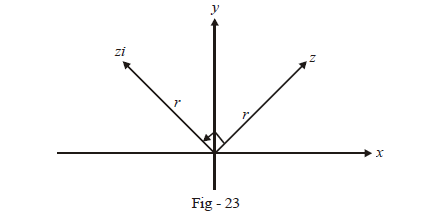### Division:

Consider two numbers $${z_1} = {x_1} + i\,{y_1}\,\,{\rm{and}}\,\,{z_2} = {x_2} + i{y_2};$$ we want to find \begin{align}\frac{{{z_1}}}{{{z_2}}}\end{align}:

$\frac{{{z_1}}}{{{z_2}}} = \frac{{{x_1} + i\,y{ _1}}}{{{x_2} + i\,{y_2}}}$

How do we reduce this expression to the standard form of a complex number $$a + i b?$$ For that, we need to make the denominator real. How can this be done?

Observe the product $$(5 – i) (5 + i)$$ that we obtained earlier as 26; this gives us the hint; multiplication of $$x + iy \; by x – iy$$ results in a real number $${x^2} + {y^2}:$$

$\frac{{{z_1}}}{{{z_2}}} = \frac{{{x_1} + i{y_1}}}{{{x_2} + i{y_2}}}\,\, \times \,\,\frac{{{x_2} - i{y_2}}}{{{x_2} - i{y_2}}}$

$= \frac{{\left( {{x_1}{x_2} + {y_1}{y_2}} \right)}}{{x_2^2 + y_2^2}} + i\frac{{\left( {{x_2}{y_1} - {x_1}{y_2}} \right)}}{{x_2^2 + {y^2}}}$

which is in the standard form $$a + ib.$$

As with multiplication, we now repeat the division process with polar forms for z1 and z2.

\begin{align}z &= \frac{{{z_1}}}{{{z_2}}} = \frac{{{r_1}\left( {\cos {\theta _1} + i\sin {\theta _1}} \right)}}{{{r_2}\left( {\cos {\theta _2} + i\sin {\theta _2}} \right)}}\\\\&= \frac{{{r_1}}}{{{r_2}}}\,\,\frac{{\left( {\cos {\theta _1} + i\sin {\theta _1}} \right)}}{{\left( {\cos {\theta _2} + i\sin {\theta _2}} \right)}}\,\, \times \,\,\frac{{\left( {\cos {\theta _2} - i\sin {\theta _2}} \right)}}{{\left( {\cos {\theta _2} - i\sin {\theta _2}} \right)}}\\\\&= \frac{{{r_1}}}{{{r_2}}}\,\,\frac{{\cos {\theta _1}\cos {\theta _2} + \sin {\theta _1}\sin {\theta _2} + i\left( {\sin {\theta _1}\cos {\theta _2} - \sin {\theta _2}\cos {\theta _1}} \right)}}{{{{\cos }^2}{\theta _2} + {{\sin }^2}{\theta _2}}}\\\\&= \frac{{{r_1}}}{{{r_2}}}\left\{ {\cos \left( {{\theta _1} - {\theta _2}} \right) + i\sin \left( {{\theta _1} - {\theta _2}} \right)} \right\}\\\\&= r\left( {\cos \theta + i\sin \theta } \right)\end{align}

where   \begin{align}r = \frac{{{r_1}}}{{{r_2}}}\,\,{\rm{and}}\,\theta = {\theta _1} - {\theta _2}\end{align}

$$\Rightarrow$$ This result says that division of complex numbers causes division of individual moduli and subtraction of arguments.

For example, if $$\left| z \right| = r$$ and $$\arg \left( z \right) = \theta ,$$ the complex number  \begin{align}z' = \frac{z}{i}\end{align}  will have $$\left| {z'} \right| = \left| z \right| = r$$  and   \begin{align}\arg \left( {z'} \right) = \arg \left( {\frac{z}{i}} \right) = \arg \left( z \right) - \arg \left( i \right) = \theta - \frac{\pi }{2}.\end{align}  Geometrically, the vector z retains the same length but gets rotated clockwise by \begin{align}\frac{\pi }{2},\end{align}  when divided by $$i:$$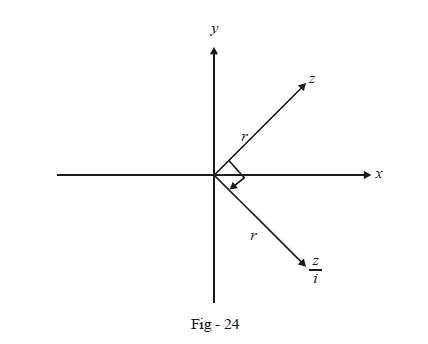The entire preceding discussion shows that arithmetic operations can be applied legitimately and consistently to complex numbers, establishing the validity of our mathematical construction of $$i.$$

In higher science and engineering courses, the reader will realise that complex numbers are indispensable.

Example- 5

Show the following operations graphically:

 (a) $$\left( {1 + i} \right)\,\, \times \,\,\left( {1 - i} \right)$$ (b) $$\left( 2 \right)\,\, \times \,\,\left( {3 + 4i} \right)$$ (c) \begin{align}\left( {\frac{3}{{13}} - \frac{4}{{13}}\,\,i} \right)\,\, \times \,\,\left( {1 + \frac{{12}}{5}\,\,i} \right)\end{align} (d) \begin{align}\frac{{2 + i}}{{2 - i}}\end{align} (e) \begin{align}\frac{{3 + 4i}}{2}\end{align} (f) \begin{align}\frac{{ - \,4 + 3i}}{{3\, + \,4i}}\end{align}

Solution:   (a)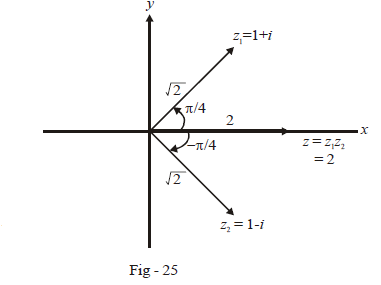\begin{align}&\left| {{z_1}} \right| = \left| {1 + i} \right| = \sqrt 2 \\&\text{arg} \left( {{z_1}} \right) = \pi /4\\ &\left| {{z_2}} \right| = \left| {1 - i} \right| = \sqrt 2 \\&\arg \left( {{z_1}} \right) = - \pi /4\Rightarrow \left| z \right| = \left| {{z_1}} \right|\left| {{z_2}} \right| = 2\\&\arg \left( z \right) = \arg \left( {{z_1}} \right) + \arg \left( {{z_2}} \right) = 0\end{align}

(b)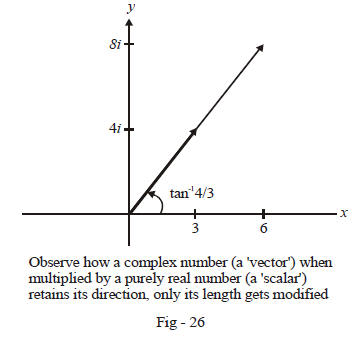\begin{align}&\left| {{z_1}} \right| = \left| 2 \right| = 2\\&\arg \left( {{z_1}} \right) = 0\\&\left| {{z_2}} \right| = \left| {3 + 4i} \right| = 5\\&\arg \left( {{z_2}} \right) = {\tan ^{ - 1}}\frac{4}{3}\\&\Rightarrow \left| z \right| = \left| {{z_1}} \right|\left| {{z_2}} \right| = 10\\&\arg \left( z \right) = \arg \left( {{z_1}} \right) + \arg \left( {{z_i}} \right)\\&= {\tan ^{ - 1}}\frac{4}{3}\end{align}

(c)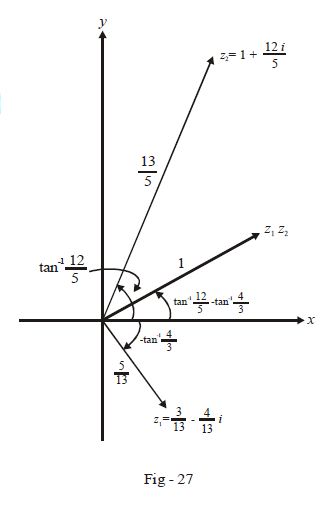\begin{align}&\left| {{z_1}} \right| = \left| {\frac{3}{{13}} - \frac{{4i}}{{13}}} \right| = \frac{5}{{13}}\\&\arg \left( {{z_1}} \right) = \arg \left( {\frac{3}{{13}} - \frac{{4i}}{{13}}} \right) = - {\tan ^{ - 1}}\left( {\frac{4}{3}} \right)\\&\left| {{z_2}} \right| = \left| {1 + \frac{{12}}{5}i} \right| = \frac{{13}}{5}\\&\arg \left( {{z_2}} \right) = \arg \left( {1 + \frac{{12i}}{5}} \right) = {\tan ^{ - 1}}\left( {\frac{{12}}{5}} \right)\\&\Rightarrow \,\,\left| z \right| = \left| {{z_1}} \right|\left| {{z_2}} \right| = 1\\&\arg \left( z \right) = \arg \left( {{z_1}} \right) + \arg \left( {{z_2}} \right)\\& = {\tan ^{ - 1}}\frac{{12}}{5} - {\tan ^{ - 1}}\frac{4}{3}\end{align}

(d)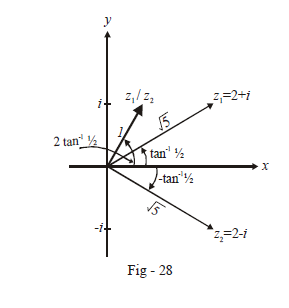\begin{align}&\left| {{z_1}} \right| = \left| {2 + i} \right| = \sqrt 5 \\&\arg \left( {{z_1}} \right) = {\tan ^{ - 1}}\frac{1}{2}\\&\left| {{z_2}} \right| = \left| {2 - i} \right| = \sqrt 5 \\&\arg \left( {{z_2}} \right) = - {\tan ^{ - 1}}\frac{1}{2}\\&\Rightarrow \left| z \right| = \frac{{\left| {{z_1}} \right|}}{{\left| {{z_2}} \right|}} = 1\\&\arg \left( z \right) = \arg \left( {{z_1}} \right) - \arg \left( {{z_2}} \right)\\&= 2{\tan ^{ - 1}}\frac{1}{2}.\end{align}

(e)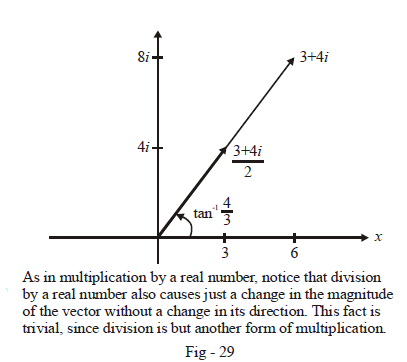\begin{align}&\left| {{z_1}} \right| = \left| {3 + 4i} \right| = 5\\&\arg \left( {{z_1}} \right) = {\tan ^{ - 1}}\frac{4}{3}\\&\left| {{z_2}} \right| = 2\\&\arg \left( {{z_2}} \right) = 0\\&\Rightarrow \left| z \right| = \frac{{\left| {{z_1}} \right|}}{{\left| {{z_2}} \right|}} = \frac{5}{2}\\&\arg \left( z \right) = \arg \left( {{z_1}} \right) - \arg \left( {{z_2}} \right)\\& = {\tan ^{ - 1}}4/3\end{align}

(f)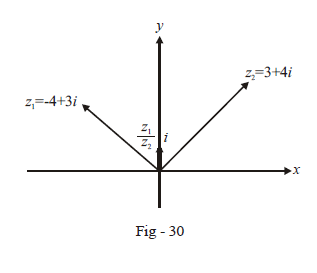Notice that  $$\left( { - 4 + 3i} \right) = i\left( {3 + 4i} \right)$$  so that

$\frac{{ - 4 + 3i}}{{3 + 4i}} = i$

TRY YOURSELF - III

Q.1  This question is for you to practice multiplication and division of complex numbers graphically. In each case, you are expected to perform the indicated operations graphically on the Argand plane.

 (a)  \begin{align}\left( {2 + 3i} \right) \times \frac{i}{{\sqrt {13} }}\end{align} (b) $$\left( { - 1 + 2i} \right) \times 3i$$ (c)  $$i \times i \times i \times i$$ (d) $$\left( {5 + i} \right) \times \left( {2 + 3i} \right)$$ (e)  \begin{align}\frac{{2 + 5i}}{{2 - 5i}}\end{align} (f)  \begin{align}\frac{{1 + 3i}}{{3 + 5i}}\end{align} (g) $$\left( {(1 \div i) \div i) \div i} \right) \div i$$ (h) \begin{align}\left( {1 + 4i} \right) \times \frac{i}{{\sqrt {17} }} \div \left( { - \frac{1}{2} + \frac{{i\sqrt 3 }}{2}} \right)\end{align}
Learn from the best math teachers and top your exams

• Live one on one classroom and doubt clearing
• Practice worksheets in and after class for conceptual clarity
• Personalized curriculum to keep up with school